# Lesson 29 Trigonometric Functions Pre Calculus Santowski 1162022

• Slides: 22Lesson 29 – Trigonometric Functions Pre. Calculus - Santowski 1/16/2022 Pre. Calculus - Santowski 1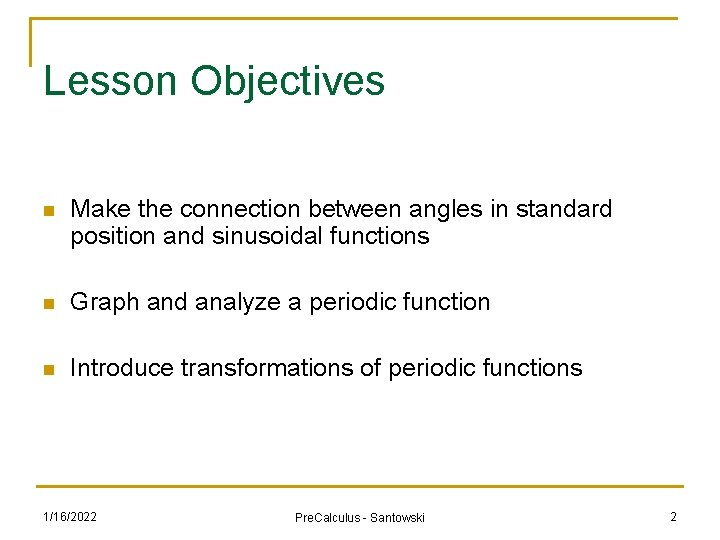Lesson Objectives n Make the connection between angles in standard position and sinusoidal functions n Graph and analyze a periodic function n Introduce transformations of periodic functions 1/16/2022 Pre. Calculus - Santowski 2(A) Key Terms Related to Periodic Functions n Define the following key terms that relate to trigonometric functions: n (a) period (b) amplitude (c) axis of the curve (or equilibrium axis) (d) domain (e) range (f) roots (g) maximum/minimum n n n 1/16/2022 IB Pre. Calculus Math SL 1 - Santowski 3(A) Key Terms 1/16/2022 IB Pre. Calculus Math SL 1 - Santowski 4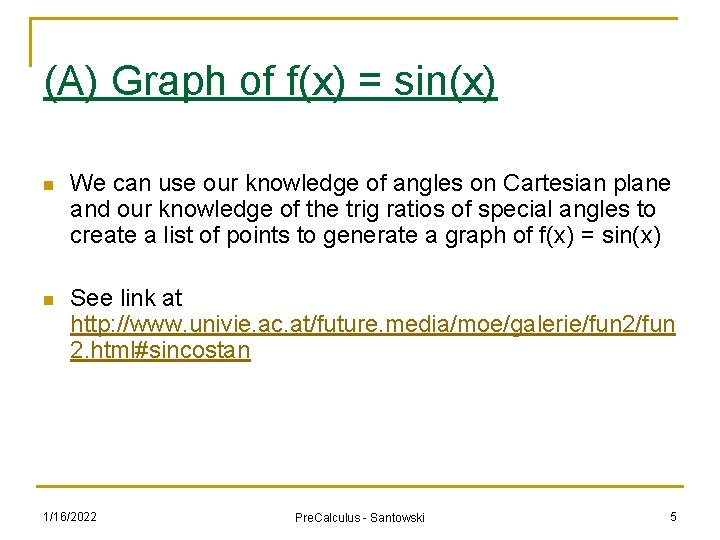(A) Graph of f(x) = sin(x) n We can use our knowledge of angles on Cartesian plane and our knowledge of the trig ratios of special angles to create a list of points to generate a graph of f(x) = sin(x) n See link at http: //www. univie. ac. at/future. media/moe/galerie/fun 2. html#sincostan 1/16/2022 Pre. Calculus - Santowski 5(A) Graph of f(x) = sin(x) 1/16/2022 Pre. Calculus - Santowski 6(A) Features of f(x) = sin(x) n n n n n The graph is periodic (meaning that it repeats itself) Domain: Range: Period: length of one cycle, how long does the pattern take before it repeats itself . x-intercepts: Axis of the curve or equilibrium axis: amplitude: max height above equilibrium position - how high or low do you get y-intercept: max. points: min. points: 1/16/2022 Pre. Calculus - Santowski 7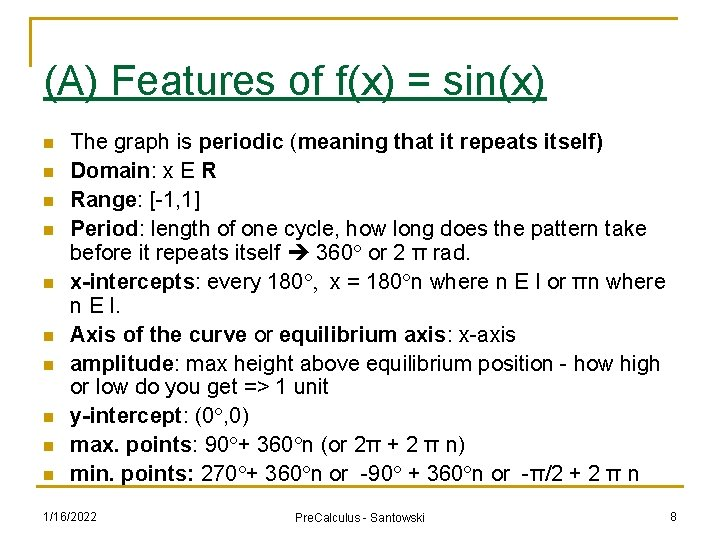(A) Features of f(x) = sin(x) n n n n n The graph is periodic (meaning that it repeats itself) Domain: x E R Range: [-1, 1] Period: length of one cycle, how long does the pattern take before it repeats itself 360° or 2 π rad. x-intercepts: every 180°, x = 180°n where n E I or πn where n E I. Axis of the curve or equilibrium axis: x-axis amplitude: max height above equilibrium position - how high or low do you get => 1 unit y-intercept: (0°, 0) max. points: 90°+ 360°n (or 2π + 2 π n) min. points: 270°+ 360°n or -90° + 360°n or -π/2 + 2 π n 1/16/2022 Pre. Calculus - Santowski 8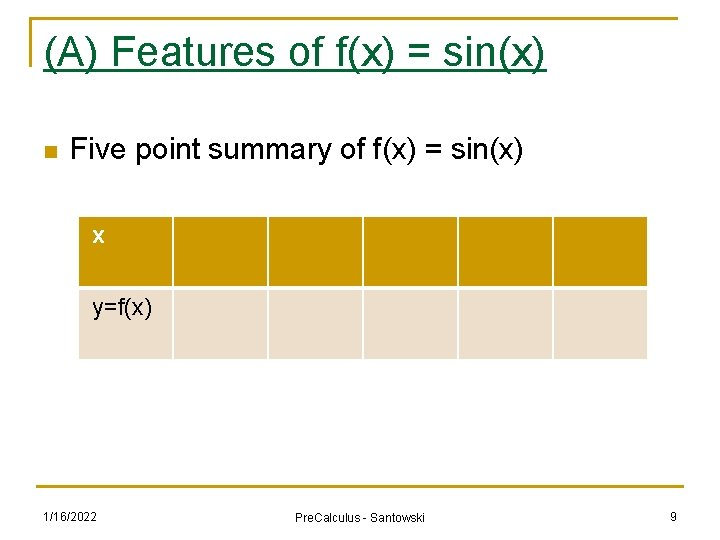(A) Features of f(x) = sin(x) n Five point summary of f(x) = sin(x) x y=f(x) 1/16/2022 Pre. Calculus - Santowski 9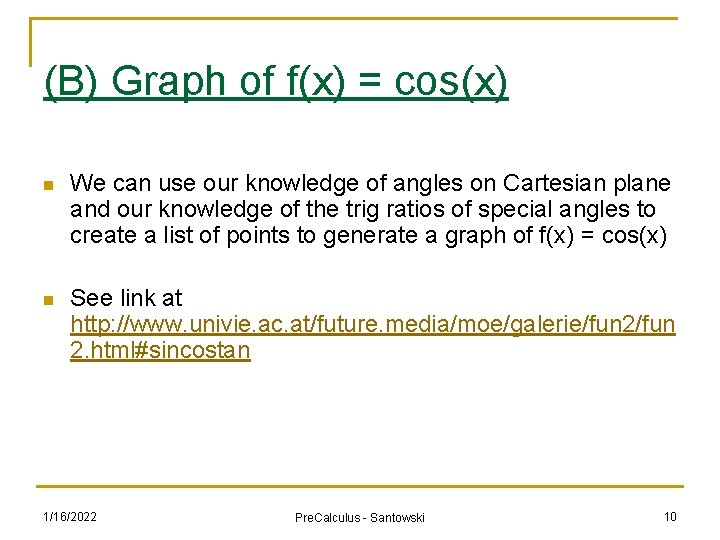(B) Graph of f(x) = cos(x) n We can use our knowledge of angles on Cartesian plane and our knowledge of the trig ratios of special angles to create a list of points to generate a graph of f(x) = cos(x) n See link at http: //www. univie. ac. at/future. media/moe/galerie/fun 2. html#sincostan 1/16/2022 Pre. Calculus - Santowski 10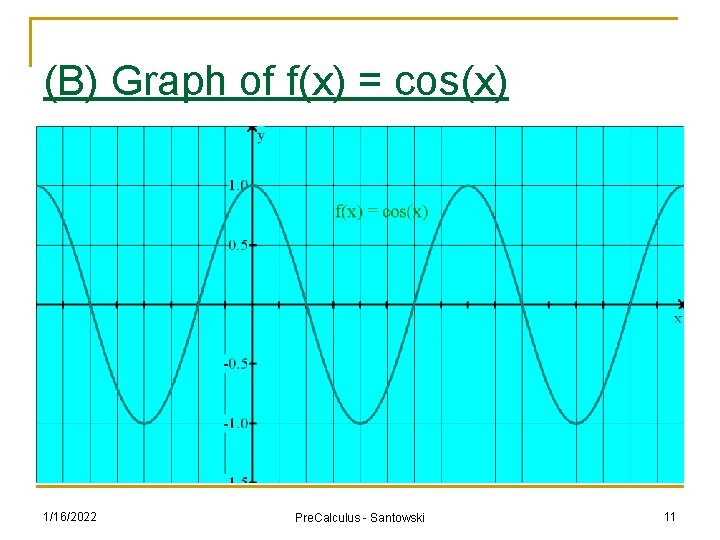(B) Graph of f(x) = cos(x) 1/16/2022 Pre. Calculus - Santowski 11(B) Features of f(x) = cos(x) n n n n n The graph is periodic Domain: Range: Period: length of one cycle, how long does the pattern take before it repeats itself . x-intercepts: Axis of the curve or equilibrium axis: amplitude: max height above equilibrium position - how high or low do you get y-intercept: max. points: min. points: 1/16/2022 Pre. Calculus - Santowski 12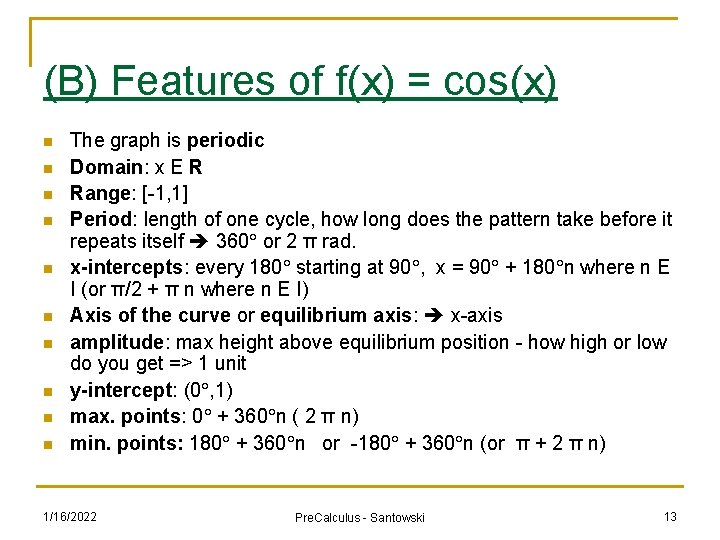(B) Features of f(x) = cos(x) n n n n n The graph is periodic Domain: x E R Range: [-1, 1] Period: length of one cycle, how long does the pattern take before it repeats itself 360° or 2 π rad. x-intercepts: every 180° starting at 90°, x = 90° + 180°n where n E I (or π/2 + π n where n E I) Axis of the curve or equilibrium axis: x-axis amplitude: max height above equilibrium position - how high or low do you get => 1 unit y-intercept: (0°, 1) max. points: 0° + 360°n ( 2 π n) min. points: 180° + 360°n or -180° + 360°n (or π + 2 π n) 1/16/2022 Pre. Calculus - Santowski 13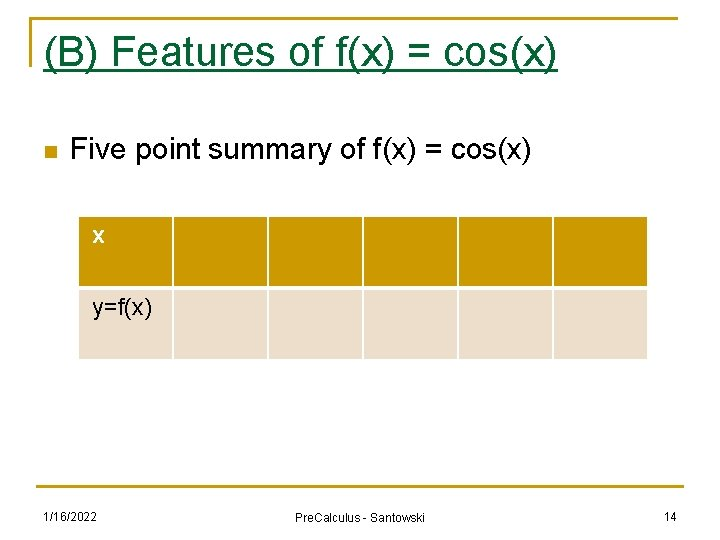(B) Features of f(x) = cos(x) n Five point summary of f(x) = cos(x) x y=f(x) 1/16/2022 Pre. Calculus - Santowski 14(C) Graph of f(x) = tan(x) n We can use our knowledge of angles on Cartesian plane and our knowledge of the trig ratios of special angles to create a list of points to generate a graph of f(x) = tan(x) n See link at http: //www. univie. ac. at/future. media/moe/galerie/fun 2. html#sincostan 1/16/2022 Pre. Calculus - Santowski 15(C) Graph of f(x) = tan(x) 1/16/2022 Pre. Calculus - Santowski 16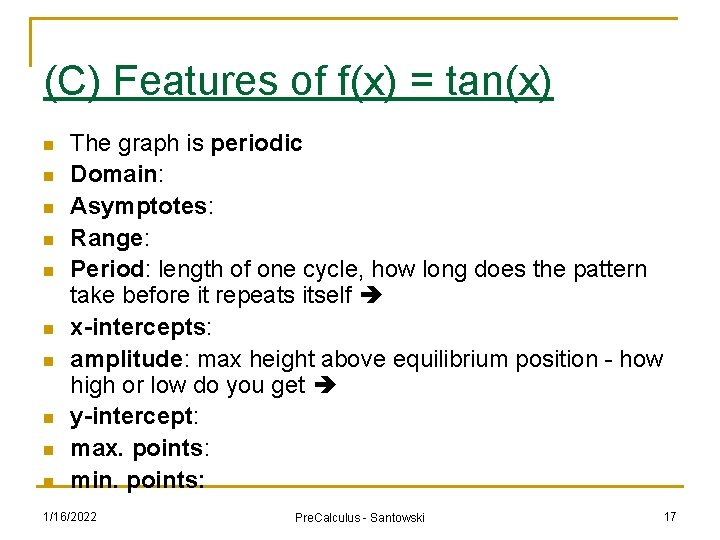(C) Features of f(x) = tan(x) n n n n n The graph is periodic Domain: Asymptotes: Range: Period: length of one cycle, how long does the pattern take before it repeats itself x-intercepts: amplitude: max height above equilibrium position - how high or low do you get y-intercept: max. points: min. points: 1/16/2022 Pre. Calculus - Santowski 17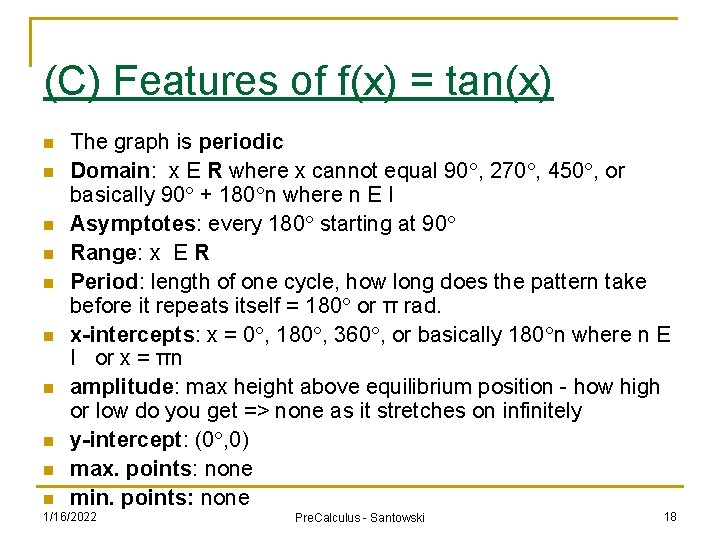(C) Features of f(x) = tan(x) n n n n n The graph is periodic Domain: x E R where x cannot equal 90°, 270°, 450°, or basically 90° + 180°n where n E I Asymptotes: every 180° starting at 90° Range: x E R Period: length of one cycle, how long does the pattern take before it repeats itself = 180° or π rad. x-intercepts: x = 0°, 180°, 360°, or basically 180°n where n E I or x = πn amplitude: max height above equilibrium position - how high or low do you get => none as it stretches on infinitely y-intercept: (0°, 0) max. points: none min. points: none 1/16/2022 Pre. Calculus - Santowski 18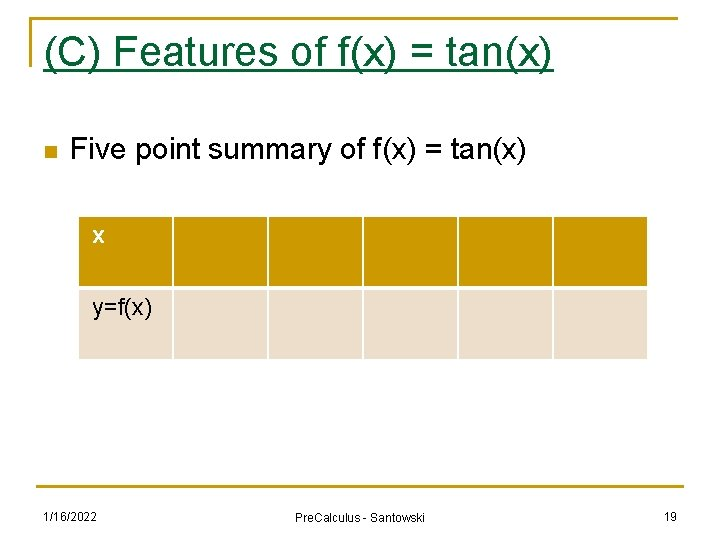(C) Features of f(x) = tan(x) n Five point summary of f(x) = tan(x) x y=f(x) 1/16/2022 Pre. Calculus - Santowski 19Graphs of Secondary Trig Fcns csc n Recall that csc(x) = 1/sin(x) n Here is a data table for y = sin(x) x 0 30 60 90 120 150 180 210 240 270 300 330 360 y 0 0. 5 0. 87 1 0. 87 0. 5 0 -0. 5 -0. 87 -1 -0. 87 -0. 5 0 n How would you prepare a graph for y = csc(x)? 1/16/2022 Pre. Calculus - Santowski 20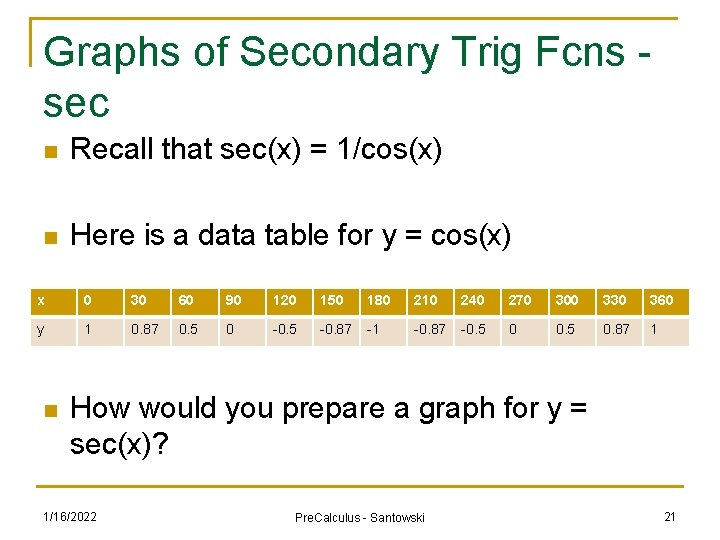Graphs of Secondary Trig Fcns sec n Recall that sec(x) = 1/cos(x) n Here is a data table for y = cos(x) x 0 30 60 90 120 150 180 210 240 270 300 330 360 y 1 0. 87 0. 5 0 -0. 5 -0. 87 -1 -0. 87 -0. 5 0. 87 1 n How would you prepare a graph for y = sec(x)? 1/16/2022 Pre. Calculus - Santowski 21Graphs of Secondary Trig Fcns cot n Recall that cot(x) = 1/tan(x) n Here is a data table for y = tan(x) x 0 30 60 90 120 150 180 210 240 270 300 330 360 y 0 0. 57 1. 73 Und. -1. 73 -0. 57 0 n How would you prepare a graph for y = cot(x)? 1/16/2022 Pre. Calculus - Santowski 22